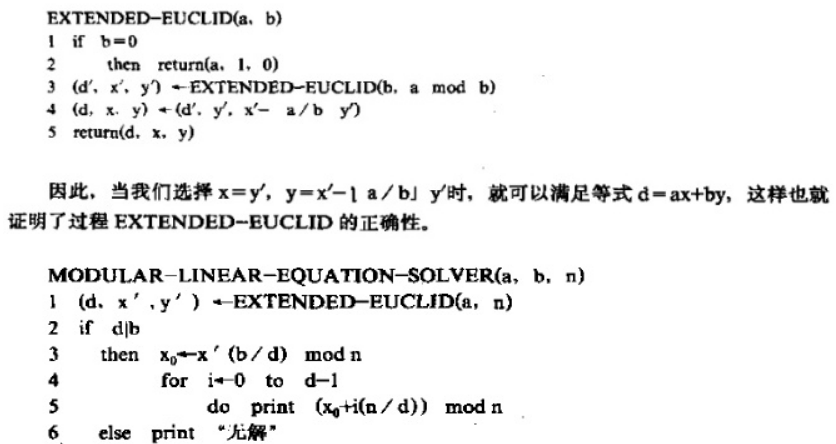# POJ 2115 - C Looooops

## 解题思路

`i=65534`，当 `i+=3` 时，`i=1`

`x = [(B-A+2^k)%2^k] / C`

`Cx=(B-A)(mod 2^k)` 此方程为 模线性方程，本题就是求X的值。

• `a = C`
• `b = B-A`
• `n = 2^k`

`ax = b (mod n)`

`d = gcd(a,n)`• 计算 `n=2^k` 时，用位运算是最快的，`1<<k` （1左移k位）就是 `2^k`
• 但是使用 `long long` 的同学要注意格式， `1LL<<k`
• 使用 `__int64` 的同学要强制类型转换 `(__int64)1<<k`

## AC 源码

``````//Memory Time
//212K   0MS

#include<iostream>
using namespace std;

//d=ax+by,其中最大公约数d=gcd(a,n)，x、y为方程系数，返回值为d、x、y
__int64 EXTENDED_EUCLID(__int64 a,__int64 b,__int64& x,__int64& y)
{
if(b==0)
{
x=1;
y=0;
return a;  //d=a，x=1,y=0,此时等式d=ax+by成立
}
__int64 d=EXTENDED_EUCLID(b,a%b,x,y);
__int64 xt=x;
x=y;
y=xt-a/b*y;  //系数x、y的取值是为满足等式d=ax+by
return d;
}

int main(void)
{
__int64 A,B,C,k;
while(scanf("%I64d %I64d %I64d %I64d",&A,&B,&C,&k))
{
if(!A && !B && !C && !k)
break;

__int64 a=C;
__int64 b=B-A;
__int64 n=(__int64)1<<k;  //2^k
__int64 x,y;
__int64 d=EXTENDED_EUCLID(a,n,x,y);  //求a,n的最大公约数d=gcd(a,n)和方程d=ax+by的系数x、y

if(b%d!=0)  //方程 ax=b(mod n) 无解
cout<<"FOREVER"<<endl;
else
{
x=(x*(b/d))%n;  //方程ax=b(mod n)的最小解
x=(x%(n/d)+n/d)%(n/d);  //方程ax=b(mod n)的最整数小解
printf("%I64d\n",x);
}
}
return 0;
}``````

目录